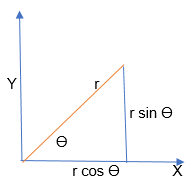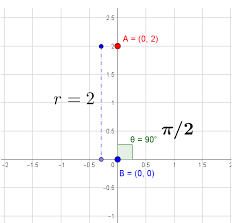# Types of Coordinate Systems

A coordinate system is used to determine each point uniquely in a plane. Study of the coordinate system led to innovations and research in the field of Coordinate geometry. This study was initially developed by the French Philosopher and Mathematician Rene Descartes.

We know that a number line is a line that is used to represent the integers in both positive and negative directions from the origin. Rene Descartes invented the idea of placing two such lines perpendicular to each other on a plane and locating points on the plane by referring them to these lines. Though the two perpendicular lines can be in any direction, we usually choose one line in the vertical axis and the other perpendicular to it in the horizontal axis. In this article we mainly discuss two types of coordinate systems.

## Different Types of Coordinate Systems

We have mainly two types of coordinate systems as listed below:

### Cartesian coordinate system

As stated above, it uses the concept of mutually perpendicular lines to denote the coordinate of a point.  To locate the position of a point in a plane using two perpendicular lines, we use the cartesian coordinate system. Points are represented in the form of coordinates (x, y) in two-dimension with respect to x- and y- axes.

The x-coordinate of a point is its perpendicular distance from the y-axis measured along the x-axis and it is known as Abscissa. The y-coordinate of a point is its perpendicular distance from the x-axis measured along the y-axis and it is known as Ordinate.

### Polar Coordinate System

Here, a point is chosen as the pole and a ray from this point is taken as the polar axis. Basically, we have two parameters namely angle and radius. The angle Ɵ with the polar axis has a single line through the pole measured anti-clockwise from the axis to the line.

The point will have a unique distance from the origin (r). Thus, a point in Polar coordinate system is represented as a pair of coordinates (r, Ɵ). The pole is represented by (0, Ɵ) for any value of Ɵ, where r = 0.

(r, Ɵ), (r, Ɵ + 2π) and (-r, Ɵ + π) are all polar coordinates for the same point.

The distance from the pole is called the radial coordinate, radial distance or simply radius and the angular coordinate, polar angle or azimuth.

Consider the figure below that depicts the relationship between polar and cartesian coordinates.

X = r cos Ɵ and y = r sin Ɵ

r = (x2 + y2) ½ and tan Ɵ = (y/x)Polar equation of a curve consists of points of the form (r, Ɵ).

 In case of circle, the general equation for a circle with centre at (R, β) and radius a is r2 – 2rR cos(Ɵ – β) + R2 = a2.

Radial lines (those running through the pole) are represented by the equation: Ɵ = β.

The conic sections with one focus on the pole and the other somewhere on the 0-degree ray so that the conic’s major axis lies along the polar axis is given by r = l/(1 – e cosƟ), where e is the eccentricity and is the semi-latus rectum (the perpendicular distance at a focus from the major axis to the curve).

Special Cases:

1. If e > 1, this equation defines a hyperbola; if e = 1, it defines a parabola; and

2. If e < 1, it defines an ellipse.

3. There is a special case when e = 0, it defines a circle of the radius.

## Cartesian Formulae for the Plane

Distance between two points

The distance between two points of the plane (x1, y1) and (x2, y2) is given by

d = [(x2 – x1)2 + (y2 – y1)2 ]1/2

In case of three-dimensional system, the distance formula between the points (x1, y1, z1) and (x2, y2, z2) is

d = [(x2 – x1)2 + (y2 – y1)2 + (z2 – z1)2 ]1/2

Representation of a vector

In two-dimensions, the vector from the origin to the point with the cartesian coordinates (x, y) can be written as r = xi + yj where i = (1,0) and j = (0,1) are unit vectors in the direction of the x-axis and y-axis respectively.

In case of three-dimensions, we will have r = xi + yj + zk, where k = (0,0,1) is the unit vector in the direction of z-axis.

Three dimensional geometry

Distance formula

## Example Problems

Example 1: In a graph paper, plot the point where Ajay has kept the lamp. He describes the situation as given below: the lamp is kept on a table which is taken as the reference. The lamp has a height of 2 units and is kept at the edge of the beginning end of the table.

Solution: As per the statement given in the question, the reference table is to be considered as the origin (0, 0). The position of lamp as stated above clearly points out to be on the coordinate (0, 2). Thus, the point marked A is the position of the lamp with respect to the table reference marked as B. The following graph illustrates this.Example 2: The cartesian coordinates of a point in the x-y plane is (x, y) = (-3.5, -2.5). Find the polar coordinates of this point?

Solution: We have (x, y) = (-3.5, -2.5). Thus, in polar coordinates, we have r = (x2 + y2) ½

and tan Ɵ = (y/x) = (-2.5/-3.5) = 0.714

=> Ɵ = 216 degrees (sign is based on quadrant).

Example 3: Find the distance between the two points (4,5,6) and (9,8,2) in the x-y plane?

Solution: d = [(x2 – x1)2 + (y2 – y1)2 + (z2 – z1)2 ]1/2

Given: (x1, y1, z1) = (4,5,6) and (x1, y1, z1) = (9, 8, 2)

Therefore, x2 – x1 = 9 – 4 = 5.

Similarly, y2 – y1 = 8 – 5 = 3 and z2 – z1 = 2 – 6 = -4

Hence, d2 = 52 + 32 + (-4)2

or, d = √50 units.

Example 4: The points $\left( \frac{a}{\sqrt{3}},a \right),\ \left( \frac{2a}{\sqrt{3}},\,2a \right),\ \left( \frac{a}{\sqrt{3}},\,3a \right)$ are the vertices of
A) An equilateral triangle

B) An isosceles triangle

C) A right-angled triangle

D) None of these

Solution:

Let $A\,\left( \frac{a}{\sqrt{3}},\,a \right),\,\,B\,\left( \frac{2a}{\sqrt{3}},\,\,2a \right)$ and $C\,\left( \frac{a}{\sqrt{3}},\,\,3a \right)\\$

Then $A{{B}^{2}}={{\left( \frac{a}{\sqrt{3}}-\frac{2a}{\sqrt{3}} \right)}^{2}}+{{(a-2a)}^{2}}=\frac{{{a}^{2}}}{3}+{{a}^{2}}=\frac{4{{a}^{2}}}{3}\\$

Similarly $B{{C}^{2}}=\frac{4{{a}^{2}}}{3} \text and \ A{{C}^{2}}=4{{a}^{2}}\\$

Hence it is an isosceles triangle.

Example 5: If the point (x, y) be equidistant from the points $(a+b,\,b-a)$ and $(a-b,\,a+b),$ then
$A) ax+by=0\\ B)ax-by=0\\ C)bx+ay=0\\ D)bx-ay=0\\$

Solution:

${{\left\{ x-(a+b) \right\}}^{2}}+{{\left\{ y-(b-a) \right\}}^{2}}\\={{\left\{ x-(a-b) \right\}}^{2}}+{{\left\{ y-(a+b) \right\}}^{2}} \\\Rightarrow \,\,{{x}^{2}}+{{(a+b)}^{2}}-2x\,(a+b)+{{y}^{2}}+{{(b-a)}^{2}}-2y(b-a) \\={{x}^{2}}+{{(a-b)}^{2}}-2x(a-b)+{{y}^{2}}+{{(a+b)}^{2}}-2y(a+b)\\$

On simplification, we get bx – ay = 0

Trick: The locus will be the right bisector of the line joining the given points, therefore the line must pass through the mid-points of the given point i.e. (a, b). Obviously, the line given in option (d) passes through (a, b).